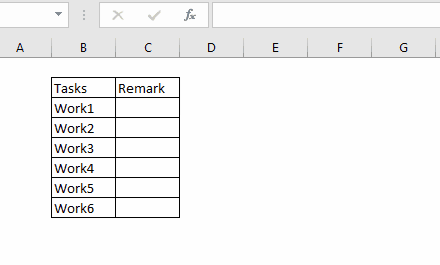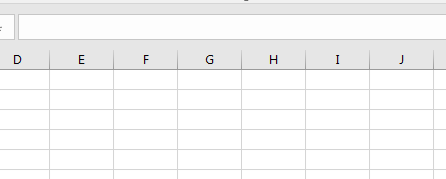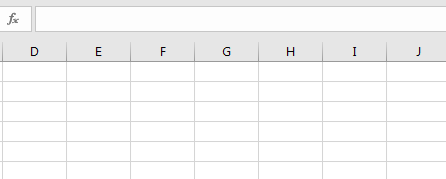# Create VBA Function to Return ArrayAs the title suggests, we will learn how to create a user-defined function in Excel that returns an array. We have already learned how to create a user-defined function in VBA. So without wasting any time let's get started with the tutorial.

What is an Array Function?

Array functions are the functions that return an array when used. These function are used with CTRL+SHIFT+ENTER key combinations and this is why we prefer calling array function or formulas as CSE function and formulas.

The excel array function is often multicell array formulas. One example is the TRANSPOSE function.

Creating a UDF array function in VBA

So, the scenario is that I just want to return the first 3 even numbers using function ThreeEven() function.

The code will look like this.

```Function ThreeEven() As Integer()

'define array
Dim numbers(2) As Integer

'Assign values to array
numbers(0) = 0
numbers(1) = 2
numbers(2) = 4

'return values
ThreeEven = numbers

End Function
```

Let us use this function on the worksheet.You can see that, we first select three cells (horizontally, for vertical we have to use two-dimensional array. We have it covered below.). Then we start writing our formula. Then we hit CTRL+SHIFT+ENTER. This fills the selected cells with the array values.

Note:

• In practice, you will not know how many cells you gonna need. In that case always select more cells than expected array length. The function will fill the cells with array and extra cells will show #N/A error.
• By default, this array function returns values in a horizontal array. If you try to select vertical cells, all cells will show the first value of array only.

How it works?

To create an array function you have to follow this syntax.

```Function functionName(variables) As returnType()

dim resultArray(length) as dataType

'Assign values to array here

functionName =resultArray

End Function
```

The function declaration must be as defined above. This declared that it is an array function.
While using it on the worksheet, you have to use CTRL+SHIFT+ENTER key combination. Otherwise, it will return the first value of the array only.

VBA Array Function to Return Vertical Array

To make your UDF array function work vertically, you don't need to do much. Just declare the arrays as a two-dimensional array. Then in first dimension add the values and leave the other dimension blank. This is how you do it:

```Function ThreeEven() As Integer()

'define array
Dim numbers(2,0) As Integer

'Assign values to array
numbers(0,0) = 0
numbers(1,0) = 2
numbers(2,0) = 4

'return values
ThreeEven = numbers

End Function
```

This is how you use it on the worksheet.

##UDF Array Function with Arguments in Excel

In the above examples, we simply printed sum static values on the sheet. Let's say we want our function to accept a range argument, perform some operations on them and return the resultant array.

Example Add "-done" to every value in the range

Now, I know this can be done easily but just to show you how you can use user-defined VBA array functions to solve problems.

So, here I want an array function that takes a range as an argument and adds "-done" to every value in range. This can be done easily using the concatenation function but we will use an array function here.

```Function CONCATDone(rng As Range) As Variant()
Dim resulArr() As Variant

'Create a collection
Dim col As New Collection

On Error Resume Next
For Each v In rng
Next
On Error GoTo 0

'completing operation on each value and adding them to Array
ReDim resulArr(col.Count - 1, 0)
For i = 0 To col.Count - 1
resulArr(i, 0) = col(i + 1) & "-done"
Next

CONCATDone = resulArr

End Function
```

Explanation:

The above function will accept a range as an argument and it will add "-done" to each value in range.

You can see that we have used a VBA collection here to hold the values of the array and then we have done our operation on each value and added them back on a two-dimensional array.So yeah guys, this is how you can create a custom VBA array function that can return an array. I hope it was explanatory enough. If you have any queries regarding this article, put it in the comments section below.

Related Articles:

Arrays in Excel Formul|Learn what arrays are in excel.

How to Create User Defined Function through VBA | Learn how to create user-defined functions in Excel

Using a User Defined Function (UDF) from another workbook using VBA in Microsoft Excel | Use the user-defined function in another workbook of Excel

Return error values from user-defined functions using VBA in Microsoft Excel | Learn how you can return error values from a user-defined function

Popular Articles:

50 Excel Shortcuts to Increase Your Productivity

The VLOOKUP Function in Excel

COUNTIF in Excel 2016

How to Use SUMIF Function in Excel

Terms and Conditions of use

The applications/code on this site are distributed as is and without warranties or liability. In no event shall the owner of the copyrights, or the authors of the applications/code be liable for any loss of profit, any problems or any damage resulting from the use or evaluation of the applications/code.